# HSSlive: Plus One & Plus Two Notes & Solutions for Kerala State Board

## AP Board Class 7 Maths Chapter 3 Simple Equations Ex 3.4 Textbook Solutions PDF: Download Andhra Pradesh Board STD 7th Maths Chapter 3 Simple Equations Ex 3.4 Book AnswersAP Board Class 7 Maths Chapter 3 Simple Equations Ex 3.4 Textbook Solutions PDF: Download Andhra Pradesh Board STD 7th Maths Chapter 3 Simple Equations Ex 3.4 Book Answers

## Andhra Pradesh State Board Class 7th Maths Chapter 3 Simple Equations Ex 3.4 Books Solutions

 Board AP Board Materials Textbook Solutions/Guide Format DOC/PDF Class 7th Subject Maths Chapters Maths Chapter 3 Simple Equations Ex 3.4 Provider Hsslive

2. Click on the Andhra Pradesh Board Class 7th Maths Chapter 3 Simple Equations Ex 3.4 Answers.
3. Look for your Andhra Pradesh Board STD 7th Maths Chapter 3 Simple Equations Ex 3.4 Textbooks PDF.
4. Now download or read the Andhra Pradesh Board Class 7th Maths Chapter 3 Simple Equations Ex 3.4 Textbook Solutions for PDF Free.

## AP Board Class 7th Maths Chapter 3 Simple Equations Ex 3.4 Textbooks Solutions with Answer PDF Download

Find below the list of all AP Board Class 7th Maths Chapter 3 Simple Equations Ex 3.4 Textbook Solutions for PDF’s for you to download and prepare for the upcoming exams:

Question 1.
Find the height of the statue from the adjacent diagram.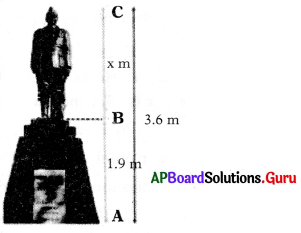From the given diagram
Height of the statue (BC) = x m
Height of the Pedastal (AB) = 1.9 m
Total Height (AC) = 3.6 m
BC + AB = 3.6 m
⇒ x + 1.9 m = 3.6 m
⇒ x + 1.9 – 1.9 = 3.6 – 1.9 (Subtract 1.9 on both sides)
⇒ x = 1.7 m
∴ Height of the statue = 1.7 m

Question 2.
The sum of twice a number and 4 is 80, find the number.
Let the number be x.
Twice the number = 2x
Sum of twice a number and 4 = 80
2x + 4 = 80
⇒ 2x + 4 – 4 = 80 – 4
(Subtract 4 on both sides)
⇒ 2x = 76
⇒ 2𝑥2 = 762 (Divide by 2 on both sides)
⇒ x = 38
∴ Number = 38

Question 3.
The difference between a number and one-fourth of itself is 24, find the number.
Let the number be a.
One-fourth of a number = 14 of a = 𝑎4
Difference of number and one-fourth of itself = 24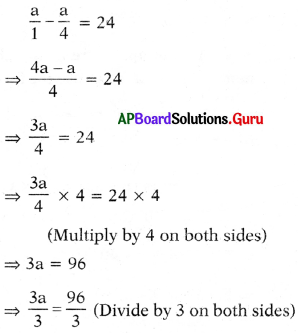⇒ a = 32
∴ The number a = 32

Question 4.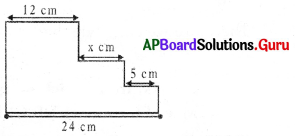Find the value of x from the adjacent diagram.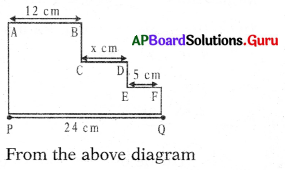From the above diagram
AB = 12 cm; CD = x cm
EF = 5 cm; PQ = 24 cm
We know,
AB + CD + EF = PQ
⇒ 12 + x + 5 = 24
⇒ x + 17 = 24
⇒ x + 17 – 17 = 24 – 17
(Subtract 17 on both sides)
⇒ x = 7 cm

Question 5.
To convert temperature from Fahrenheit to Centigrade, we use the formula (F – 32) = 95 × C. If C = – 40° C, then find F.
Given formula (F – 32) = 95 × C and C = – 40° C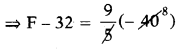⇒ F – 32 = 9(- 8)
⇒ F – 32 = – 72
⇒ F – 32 + 32 = – 72 + 32 (Add 32 on both sides)
⇒ F = – 40° F
Therefore we conclude that
– 40° C = – 40° F.

Question 6.
Rahim has ₹x, from which he spends ₹6. If twice of the money left with him is ₹86, then find x.
Rahim has money = ₹x
Money after spends ₹6 = x – 6
Twice the money left with Rahim
= 2(x – 6) = ₹86
⇒ – 2(x – 6) = 86
⇒ 2(𝑥6)2 = 862 (Divide by 2 on both sides)
⇒ x – 6 = 43
⇒ x – 6 + 6 = 43 + 6 (Add 6 on both sides)
⇒ x = 49
Therefore money at Rahim = ₹49.

Question 7.
The difference between two numbers is 7. Six times the smaller plus the larger is 77. Find the numbers.
Let the smaller number is x.
Larger number = x + 7
Six times the smaller = 6.x
Six times smaller plus the larger = 77
⇒ 6x + x + 7 = 77
⇒ 7x + 7 = 77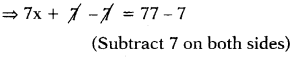⇒ 7x = 70
⇒ 7x7 = 707 (Divide by 7 on both sides) ⇒x = 10
∴ Smaller number x = 10
Larger number = x + 7 = 10 + 7 = 17
∴ The numbers are 10 and 17.

Question 8.
The sum of three consecutive even numbers is 54. Find the numbers.
Let the three consecutive even numbers are 2x, 2(x +1), 2(x + 2).
Sum of three consecutive even numbers = 54
⇒ 2x + 2(x + 1) + 2(x + 2) = 54
⇒ 2x + 2x + 2 + 2x + 4 = 54
⇒ 6x + 6 = 54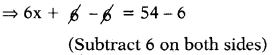⇒ 6x = 48
⇒ 6𝑥6 = 486 (Divide by 6 on both sides)
⇒ x = 8
Numbers are, 2x, 2(x + 1), 2(x + 2)
2(8), 2(8 + 1), 2(8 + 2)
∴ The numbers are 16, 18, 20.

Question 9.
In a class of 48 students, the number of girls is one third the number of boys. Find the number of girls and boys in the class.
Let the number of boys = x
The number of girls = 13 of boys
13 of x = 𝑥3
Boys + Girls = Total students
⇒ 𝑥1+𝑥3 = 48
⇒ 3𝑥+𝑥3 = 48
⇒ 4𝑥3 = 48
⇒ 4𝑥3 × 3 = 48 × 3
(Multiply by 3 on both sides)
⇒ 4x = 144
⇒ 4𝑥4 = 1444 (Divide by 4 on both sides)
⇒ x = 36
∴ Number of boys = 36
Number of girls = 𝑥3 = 363 = 12
Boys = 36 and girls = 12

Question 10.
The present ages of Mary and Joseph are in the ratio 5 : 3. After 3 years sum of their ages will be 38. Find their present ages.
Ratio of present ages of Mary and Joseph = 5:3 = 5x : 3x
After 3 years their ages
= 5x + 3 : 3x + 3
Sum of their ages = 38
⇒ 5x + 3 + 3x + 3 = 38
⇒ 8x + 6 = 38
⇒ 8x + 6 – 6 = 38 – 6 (Subtract 6 on both sides)
⇒ 8x = 32
⇒ 8𝑥8 = 328
⇒ x = 4
⇒ Ratio of present ages = 5x : 3x
= 5(4) : 3(4) = 20 : 12
Present ages of Mary and Joseph are 20 years and 12 years.

Question 11.
A sum of ₹500 is in the form of notes of denominations of ₹5 and ₹10. If the total number of notes is 90, then find the number of notes of each type.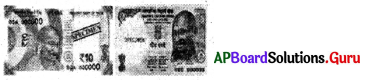Let, number of f5 notes = x
Given sum of number of ₹5 notes and ₹10 notes is 90.
So, number of ₹10 notes = 90 – x
Sum of ₹5 notes = ₹5 × x = 5x
Sum of ₹ 10 notes = ₹ 10 × (90 – x)
= 900 – 10x
⇒ 5x + 900 – 10x = 500
⇒ – 5x + 900 = 500
⇒ – 5x + 900 – 900 = 500 – 900 (Subtract 900 on both sides)
⇒ – 5x = – 400
⇒ 5𝑥5 = 4005
(Divide by – 5 on both sides)
⇒ x = 80
∴ Number of ₹5 notes x = 80
Number of ₹10 notes
= 90 – x = 90 – 80 = 10.

Question 12.
John and Ismail donated some money to the Relief Fund. The amount paid by Ismail is ₹85 more than twice that of John. If the total money paid by them is ₹4000, then find the amount of money donated by John.
Let the amount donated by John = ₹x
The amount donated by Ismail is ₹85 more than twice of John.
So, the amount donated by Ismail = ₹(2x + 85)
Total amount = John amount + Ismail amount = ₹4000
⇒ x + 2x + 85 = 4000
⇒ 3x + 85 = 4000
⇒ 3x + 85 – 85 = 4000 – 85 (Subtract 85 on both sides)
⇒ 3x = 3915
⇒ 3𝑥3 = 39153 (Divide by 3 on both sides)
⇒ x = 1305
∴ Money donated by John = x = ₹ 1305
∴ Money donated by Ismail = 2x + 85
= 2(1305) + 85
= ₹2695

Question 13.
Length of a rectangle is 4 m less than 3 times its breadth. If the perimeter of rectangle is 32 m. Then find its length and breadth.
Let breadth of a rectangle (b) = a m
Length of rectangle (l) = 4 m less than 3 times of breadth
= (3 ∙ a – 4) m
Given perimeter = 2(l + b) = 32 m
⇒ 2[3a – 4 + a] = 32
⇒ 2[4a – 4] = 32
⇒ (8a – 8) = 32 (Distributive property)
⇒ 8a – 8 + 8 = 32 + 8 (Add 8 on both sides)
⇒ 8a = 40
⇒ 8a8 = 408 (Divide by 8 on both sides) o o
⇒ a = 5
∴ breadth (b) = 5 m
length (l) = 3a – 4
= 3(5) – 4 = 15 – 4 = 11 m
∴ length = 11 m and breadth = 5 m.

Question 14.
A bag contains some number of white balls, twice the number of white balls are blue balls, thrice the number of blue balls are the red balls. If the total number of balls in the bag are 27. Then calculate the number of balls of each colour present in the bag.
Let the number of white balls = x
Number of blue balls = twice the number of white balls
= 2(x) = 2x
Number of red balls
= thrice the number of blue balls = 3(2x) = 6x
Total balls = White + Blue + Red = 27
⇒ x + 2x + 6x = 27
⇒ 9x = 27
⇒ 9𝑥9 = 279 (Divide by 9 on both sides) 9 9 .
⇒ x = 3
Therefore white balls x = 3
Blue balls = 2x = 2(3) = 6
Red balls = 6x = 6(3) = 18

Question 15.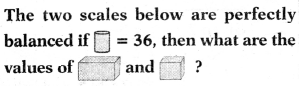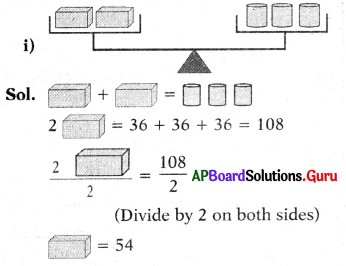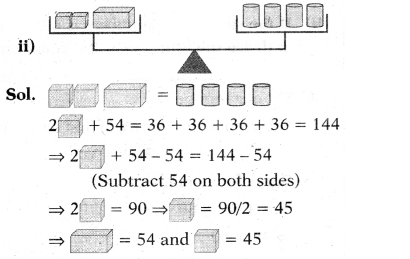## Andhra Pradesh Board Class 7th Maths Chapter 3 Simple Equations Ex 3.4 Textbooks for Exam Preparations

Andhra Pradesh Board Class 7th Maths Chapter 3 Simple Equations Ex 3.4 Textbook Solutions can be of great help in your Andhra Pradesh Board Class 7th Maths Chapter 3 Simple Equations Ex 3.4 exam preparation. The AP Board STD 7th Maths Chapter 3 Simple Equations Ex 3.4 Textbooks study material, used with the English medium textbooks, can help you complete the entire Class 7th Maths Chapter 3 Simple Equations Ex 3.4 Books State Board syllabus with maximum efficiency.

## FAQs Regarding Andhra Pradesh Board Class 7th Maths Chapter 3 Simple Equations Ex 3.4 Textbook Solutions

#### Can we get a Andhra Pradesh State Board Book PDF for all Classes?

Yes you can get Andhra Pradesh Board Text Book PDF for all classes using the links provided in the above article.

## Important Terms

Andhra Pradesh Board Class 7th Maths Chapter 3 Simple Equations Ex 3.4, AP Board Class 7th Maths Chapter 3 Simple Equations Ex 3.4 Textbooks, Andhra Pradesh State Board Class 7th Maths Chapter 3 Simple Equations Ex 3.4, Andhra Pradesh State Board Class 7th Maths Chapter 3 Simple Equations Ex 3.4 Textbook solutions, AP Board Class 7th Maths Chapter 3 Simple Equations Ex 3.4 Textbooks Solutions, Andhra Pradesh Board STD 7th Maths Chapter 3 Simple Equations Ex 3.4, AP Board STD 7th Maths Chapter 3 Simple Equations Ex 3.4 Textbooks, Andhra Pradesh State Board STD 7th Maths Chapter 3 Simple Equations Ex 3.4, Andhra Pradesh State Board STD 7th Maths Chapter 3 Simple Equations Ex 3.4 Textbook solutions, AP Board STD 7th Maths Chapter 3 Simple Equations Ex 3.4 Textbooks Solutions,
Share: